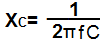﻿ What is Impedance? ﻿# What is Impedance?Impedance is the amount of resistance that a component offers to current flow in a circuit at a specific frequency.

In this article, we'll talk about how impedance is similar to and how it differs from just plain resistance.

First, we'll not the similarities of the two. Impedance, just like resistance, is a value which show the amount of resistance that a component has to the flow of electrical current. And just like resistance, the unit to measure impedance is ohms(Ω).

However, unlike resistance, impedance differs in that the amount of resistance that a component has to a signal varies with the signal's frequency. This means that the resistance of the component varies depending on the frequency of the signal entering the component. Resistance is a value and measure which is independent of frequency. It doesn't take into account the frequency of the signal passing through it, because frequency doesn't affect the resistance of nonreactive components. However, reactive components (which we'll discuss below) change the amount of resistance they offer in a circuit depending on the input signal's frequency. But impedance varies according to the frequency of the signal entering it. This is the difference between resistance and impedance.

So the next step question to answer is, which components are affected by frequency and offer different resistances based on frequency and which components do not change according to the frequency entering in?

And the answer is, nonreactive components do not care about the frequency of the signal entering into the component. They do not change resistance values based on the input frequency. One such component is the resistor, which operates independent of frequency. Whether the voltage going through it is DC or AC doesn't affect the amount of resistance it offers. It's the same for both DC and AC signals.

However, reactive components, the 2 main ones being capacitors and inductors, change resistance values based on the frequency of the signal entering into them. Capacitors are reactive devices which have high impedance at low frequencies and low impedance at higher frequencies. As the frequency increase, the reactance decreases. Inductors are devices that have low impedance at low frequencies and higher impedance at higher frequencies. As the frequency increases, the impedance increases. These are referred to as inductive reactance and capacitive reactance.

Impedance is a crucial concept to understand as most electronic circuits utilize capacitors and inductors. The main point to understand is that they are frequency dependent.

### How to Calculate Impedance

Now we will go over how to calculate the impedance of the 2 main reactive components, capacitors and inductor.

The impedance of capacitors and inductors each have separate formulas, so the correct formula needs to be applied for each one.

### Capacitor Impedance

To calculate the impedance of a capacitor, the formula to do so is:where XC is the impedance in unit ohms, f is the frequency of the signal passing through the capacitor, and C is the capacitance of the capacitor.

To use our online calculator that will calculate capacitor impedance automatically for you, visit the resource Capacitor Impedance Calculator.

### Inductor Impedance

To calculate the impedance of an inductor, the formula to do so is:where XL is the impedance in unit ohms, f is the frequency of the signal passing through the inductor, and L is the inductance of the inductor.

To use our online calculator that will calculate inductor impedance automatically for you, visit the resource Inductor Impedance Calculator.

If there are both capacitors and inductors present in a circuit, the total amount of impedance can be calculated by adding all of the individual impedances:

XTotal= XC + XL

Related Resources

Inductive Reactance

Capacitive Reactance

Source Transformation of Circuits